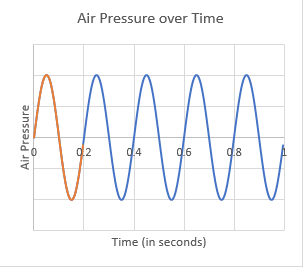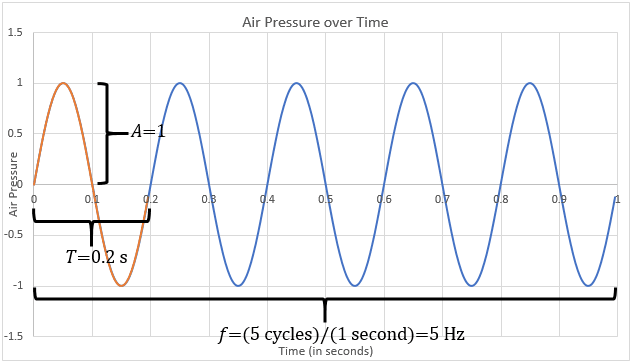# Introduction to the Sine Wave

March 29, 2018

The sine wave is fundamental to vibration testing. It is the basis of sine testing, which engineers use to identify resonances and validate fixtures. The sine wave also lies at the heart of the Fourier transform: the fundamental tool in the analysis of random waveforms.

### Frequency

Figure 1.1 shows a sine wave oscillating with regular frequency and amplitude. A wave’s frequency refers to the number of cycles—or oscillations—that occur in one second. The orange portion of the wave from 0-0.2 seconds is a single cycle.Figure 1.1. A sine wave for air pressure over time. The orange portion of the wave from 0-0.2 seconds is a single cycle.

By definition, this sound wave generated by a tuning fork is sinusoidal. Its frequency is the number of normal pressure to high pressure to normal pressure to low-pressure cycles that occur in one second.

#### Period

In Figure 1.1, the air pressure-measuring device recorded five cycles over one second. This means the tuning fork was vibrating at 5Hz, where the unit hertz (Hz) is cycles per second.

The period of a wave refers to the time it takes for the sine wave to complete a cycle. It is the inverse of frequency. If the frequency of a wave is 5Hz, then each cycle takes 1/5 of a second to complete. The period of a 5Hz sine wave is 1/5Hz, which equals 0.2 seconds.

### Amplitude

A sine wave oscillates with regular frequency and amplitude. We have learned about frequency—the number of cycles the wave completes in one second. Amplitude indicates how far the wave deviates from its position of rest.

The amplitude of the tuning fork’s sound wave would depend on how hard we struck the fork. If we struck it with greater force, the fork’s arms would move with greater amplitude, and greater pressure would move through the air.

Figure 1.2 illustrates the characteristics of a sine wave on a graph.Figure 1.2. Characteristics of a sine wave: frequency (f), amplitude (A), and period (T). The orange portion of the wave signifies a complete cycle (from 0-0.2 seconds).